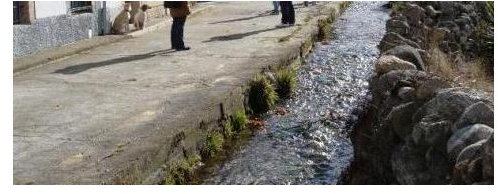# How to Model an Urban Drainage System

## Introduction

When it rains, all water in excess starts to flow along the streets. When we say “water in excess,” we are talking about the water that doesn’t infiltrate in our backyards. So, when a Hydraulic Engineer models a city’s drainage system, the most important things to calculate are: the curb’s height, number and location of sewers, and the pipe’s dimensions.

You can ask yourself: why are these parameters so important?Well, to calculate the amount of water that transits in front of your house, we must first delimit our basin, so we must find the highest elevation street point from which the water comes. Then, we have to know all the streets that contribute to the street we are modeling.

Of course there is other information we have to know before starting to model, such as: the storm's return period, the type and uses of land, population, slopes, etc. After we have all the information, we can use several software packages, such as Epa Swmm, Hec Ras, etc., to calculate the flow, velocity, and water elevation in the street. In this article, we will expand on two calculation methods: the Rational Method and the EPA SWMM software.

## Rational Method

This method is the easiest way to calculate the flow peak for a determined storm. On the other hand this method becomes too difficult to materialize in a urban basin, because we have to do circular calculations. That's because we have to limit the velocity on curbs. If this parameter exceeds 5 m/s, the curb will probably become eroded (remember that generally curbs are made of concrete, so this is the maximum velocity allowed).

The rational equation is: Q = c.i.A/360

• Q (m3/s): peak discharge flow.
• c (adimensional): runoff coeficient. It varies with the storm's return period, ground cover, and the uses of the land.
• i (mm/h): rainfall intensity.
• A (há.): drainage area.
• 360 is the coefficient that permits work with the different units in the SI System. To work in other unit system you have to do the conversions.

## EPA SWMM software

The EPA SWMM (Environmental Protection Agency Storm Water Management Model) is a dynamic rainfall-runoff simulation model used for simulation of runoff quantity from urban areas. To start modelling we first have to enter all the basin's information in each case:
• Subcatchments: area of subcatchment, average surface slope, percent of impervious area, N Manning's coefficient, Infiltration parameters, etc.
• Nodes: elevation of junction's invert, maximum water depth, etc.
• Links: conduit's cross section geometry (in the curb case is similar to a triangle), conduit length, N Manning's coefficient, energy losses, etc..
This software allows us to model all the basin, and it enables to look at depth in every node (corner, sewers), as flow hydogram, velocity and water elevation in every link (curbs, pipes). Once we model ALL the system, we’ll have to proceed in this way:
• Anytime the water elevation that transits along the curb, reaches the 85% of the curb’s height: we must place a sewer in this point.
• Anytime the water elevation in the pipe which carries water in excess, exceeds the 80% of the pipe’s height: we must increase pipe diameter to the next commercial dimension.

The heaviest work we have is to try to minimize the number of sewers and the pipe’s dimension, because these factors will economically restrict the project. To do this, we must model several number of water routes along the different streets. In this way we will choose the best route with the lowest number of sewers and pipe lengths.